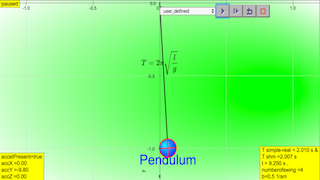shm01

## Guiding Questions

1. What are the characteristics of periodic motion? How can we study and describe such motion?
2. How can circular motion be related to simple harmonic motion (SHM)?
3. How do we analyse simple harmonic motion?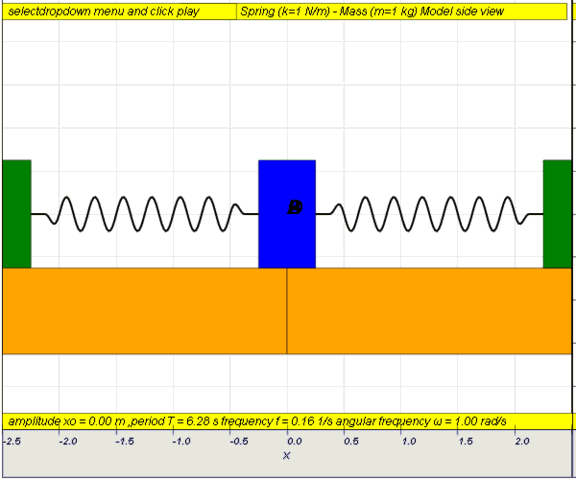### Learning Outcomes (LOs)

1. describe simple examples of free oscillations.
2. investigate the motion of an oscillator using experimental and graphical methods.
3. understand and use the terms amplitude, period, frequency and angular frequency.
4. recognise and use the equation a =  - ω2 x as the defining equation of simple harmonic motion.
5. recall and use x= x0 ω sin( ωt )as a solution to the equation a =  - ω2 x
6. recognise and use v = v0 cos ( ω t )  , $v = ±ω √(x0^2 - x^2)$
7. describe with graphical illustrations, the changes in displacement, velocity and acceleration during simple harmonic motion.
8. describe the interchange between kinetic and potential energy during simple harmonic motion.
9. describe practical examples of damped oscillations with particular reference to the effects of the degree of damping and the importance of critical damping in cases such as a car suspension system.
10. describe practical examples of forced oscillations and resonance.
11. describe graphically how the amplitude of a forced oscillation changes with driving frequency near to the natural frequency of the system, and understand qualitatively the factors which determine the frequency response and sharpness of the resonance
12. show an appreciation that there are some circumstances in which resonance is useful and other circumstances in which resonance should be avoided.

### Learning Experiences

1. Examine representations used in oscillations
• Examine how different graphs are used to represent SHM. Draw links between the different graphs highlighting the positive and negative values and their interpretation. These will help students to understand SHM as a function of two variables (space and time) and understand the treatment of vector and scalar quantities .
2. Investigate factors affecting period of oscillations using experiments or a simulation
• Design and carry out an experiment  to build a pendulum or spring-mass system to obtain a prescribed period. Present the experimental design, explaining the factors that determine the period of the oscillating system. This provides an opportunity for students to predict and collect data to ascertain the properties of a system and determine whether a motion is considered to be SHM. Students are also to understand that it is necessary for oscillations to be small for the motion to be considered as SHM. Students can also be shown other systems that demonstrate SHM . Simulations    ,  can also be used for students to investigate these factors.
3. Investigate phase using a turntable or a simulation
• Investigate phase using a turntable  or a simulation  to associate each position in an SHM with a position on a uniform circular motion. This idea can be extended to the phase difference between different positions in an SHM. The association of SHM with uniform circular motion helps students to visualise many aspects of SHM. Students are to make mental links between three related ideas: physical position and velocity of the oscillator, angle in the associated uniform circular motion and the position of the oscillator on a displacement-time graph .
4. Build a model for SHM using a datalogger or simulation
• Investigate the motion of a SHM system (pendulum, mass-spring system) using a datalogger  or a simulation . Students are to practice observing the motion and interpreting graphs before getting into the mathematical details of the equations. Compare the relationship between the different variables particularly note the values of these valuables at equilibrium position and at endpoints. Students should practice converting one graph into another.
5. Explore modelling of oscillation in molecules as SHM
• Explore how oscillations of molecules bounded by the van der Waals interaction can be SHM if the amplitude is small  .
6. Explore benefits and danger of resonance

### 1.1 YouTube of many examples of oscillators

http://youtu.be/VKtEzKcg6_s This video can be used at the start of the first lecture (while students settle down for lecture) to introduce oscillations, to show various modes of oscillations and to interest them.

### 1.1.1 Example 1: Simple pendulum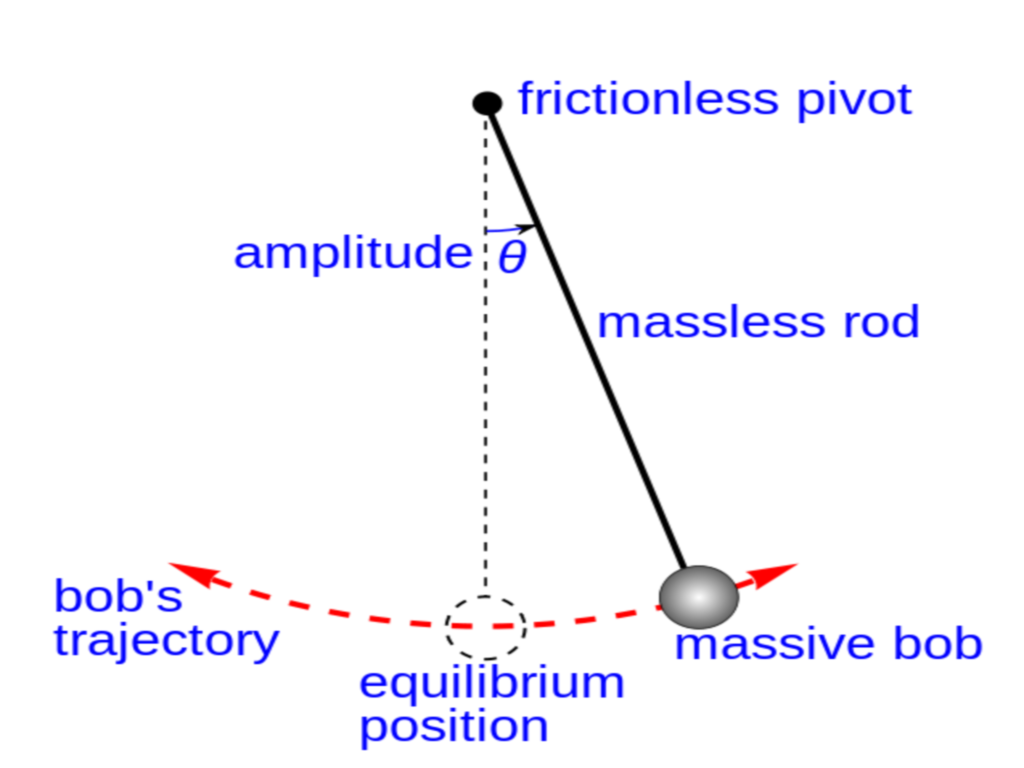Static picture of a pendulum bob given an initial horizontal displacement and released to swing freely to produce to and fro motion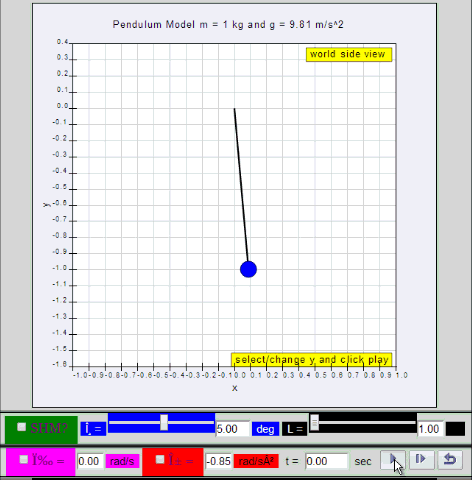Dynamic picture of a pendulum bob given an initial horizontal displacement and released to swing freely to produce to and fro motion

### 1.1.2 Run Model:

1.1.2.Q1: what is the maximum angle of release before the motion is not accurately described as a simple harmonic motion for the case of a simple free pendulum?

1.1.2.A1: : 10 degrees for error of , depending on what is the error acceptable, small angle is typically about less than 10 degree of swing from the vertical.

### Translations

Code Language Translator Run### Software Requirements

SoftwareRequirements

 Android iOS Windows MacOS with best with Chrome Chrome Chrome Chrome support full-screen? Yes. Chrome/Opera No. Firefox/ Samsung Internet Not yet Yes Yes cannot work on some mobile browser that don't understand JavaScript such as..... cannot work on Internet Explorer 9 and below

### Credits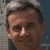This email address is being protected from spambots. You need JavaScript enabled to view it.; Fu-Kwun Hwang; Francisco Esquembre; Wolfgang Christian; Flix J. Garca

### end faq

http://iwant2study.org/lookangejss/02_newtonianmechanics_8oscillations/ejss_model_SHM01/SHM01_Simulation.xhtml

### Other Resources

1.  https://www.geogebra.org/m/sux2Q5ak The Conical Pendulum by ukukuku

## Explore some factors that affect the period of a pendulum.

Press the play button. Watch the graph to see how the angle of the pendulum changes as it swings back and forth. Use the graph to determine the period of the pendulum. Adjust the scale by dragging the numbers on the axes. Change each variable – gravity, rod length, starting angle and mass – and observe how each one affects the period. Can you explain why? Try the damping slider. Does damping change the period?

## Explore the motion of a pendulum on a spring.

Press the play button. Watch the graphs to see the motion of the spring pendulum. Can you distinguish the pattern of spring motion (up and down) from the pattern of pendulum motion (back and forth)? How are the two related? Is there a regular pattern to the motion? Compare the periods of the two graphs. How many different patterns can you produce by changing the variables – gravity, rod length, mass and spring constant? Under what conditions does the energy switch between back and forth and up and down? Does the initial starting angle affect the patterns when all the other variables are unchanged?